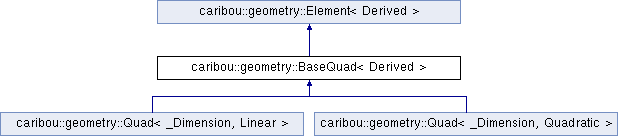Caribou
caribou::geometry::BaseQuad< Derived > Struct Template Reference
Inheritance diagram for caribou::geometry::BaseQuad< Derived >:## Public Types

using Base = Element< Derived >

using LocalCoordinates = typename Base::LocalCoordinates

using WorldCoordinates = typename Base::WorldCoordinates

using GaussNode = typename Base::GaussNode

template<UNSIGNED_INTEGER_TYPE Dim>
using Vector = typename Base::template Vector< Dim >

template<UNSIGNED_INTEGER_TYPE Rows, UNSIGNED_INTEGER_TYPE Cols>
using Matrix = typename Base::template Matrix< Rows, Cols >Public Types inherited from caribou::geometry::Element< Derived >
using Scalar = FLOATING_POINT_TYPE

using Vector = Eigen::Matrix< Scalar, Dim, 1 >

using Matrix = Eigen::Matrix< Scalar, Rows, Cols, Options >

using MatrixI = Eigen::Matrix< Scalar, Rows, Cols, Options >

using LocalCoordinates = Vector< CanonicalDimension >

using WorldCoordinates = Vector< Dimension >

## Public Member Functions

Default empty constructor.

template<typename EigenType , REQUIRES(EigenType::RowsAtCompileTime==NumberOfNodesAtCompileTime) >
Constructor from an Eigen matrix containing the positions of the quad's nodes.

template<typename EigenType , REQUIRES(EigenType::RowsAtCompileTime==NumberOfNodesAtCompileTime) >
BaseQuad (const Eigen::EigenBase< EigenType > &nodes)
Constructor from an Eigen matrix containing the positions of the quad's nodes.

template<typename ... Nodes>
BaseQuad (const WorldCoordinates &first_node, Nodes &&...remaining_nodes)
Constructor from a serie of nodes.

auto edges () const
Get the list of node indices of the edges. More...

auto frame () const -> Matrix< Dimension, Dimension >
Extract the orthogonal frame of the element by computing the cross product of the unit vectors from the center position to its projection on opposite faces. More...

auto frame (const LocalCoordinates &local_point) const -> Matrix< Dimension, Dimension >
Extract the frame positioned at the given position (in local coordinates) on the quad by computing the cross product of the unit vectors from the given position its projection on opposite edges. More...Public Member Functions inherited from caribou::geometry::Element< Derived >
auto number_of_nodes () const -> UNSIGNED_INTEGER_TYPE
Get the number of nodes in the element.

auto number_of_gauss_nodes () const -> UNSIGNED_INTEGER_TYPE
Get the number of gauss nodes in the element.

auto node (const UNSIGNED_INTEGER_TYPE &index) const
Get the Node at given index.

auto nodes () const
Get the set of nodes.

auto gauss_node (const UNSIGNED_INTEGER_TYPE &index) const -> const GaussNode &
Get the gauss node at given index.

auto gauss_nodes () const -> const std::vector< GaussNode > &
Get the set of gauss nodes.

auto number_of_boundary_elements () const
Get the number of boundary elements (ex. More...

auto boundary_elements_node_indices () const -> const auto &
Get the list of node indices of the boundary elements. More...

auto boundary_element (const UNSIGNED_INTEGER_TYPE &boundary_id) const
Construct and return the given boundary element. More...

auto L (const LocalCoordinates &xi) const -> Vector< NumberOfNodesAtCompileTime >
Get the Lagrange polynomial values evaluated at local coordinates xi w.r.t each element's interpolation nodes. More...

auto dL (const LocalCoordinates &xi) const -> Matrix< NumberOfNodesAtCompileTime, CanonicalDimension >
Get the Lagrange polynomial derivatives w.r.t the local frame {dL/du} evaluated at local coordinates {u} w.r.t each segment's interpolation nodes. More...

auto center () const -> WorldCoordinates
Get the position at the center of the element.

auto world_coordinates (const LocalCoordinates &coordinates) const -> WorldCoordinates
Get the world coordinates of a point from its local coordinates.

auto local_coordinates (const WorldCoordinates &coordinates) const -> LocalCoordinates
Get the local coordinates of a point from its world coordinates by doing a set of Newton-Raphson iterations. More...

auto local_coordinates (const WorldCoordinates &coordinates, const LocalCoordinates &starting_point, const FLOATING_POINT_TYPE &residual_tolerance, const UNSIGNED_INTEGER_TYPE &maximum_number_of_iterations) const -> LocalCoordinates
Get the local coordinates of a point from its world coordinates by doing a set of Newton-Raphson iterations. More...

auto contains_local (const LocalCoordinates &xi, const FLOATING_POINT_TYPE &eps=1e-10) const -> bool
Return true if the element contains the point located at the given local coordinates. More...

auto interpolate (const LocalCoordinates &coordinates, const Eigen::MatrixBase< MatrixType > &values) const
Interpolate a value at local coordinates from the given interpolation node values. More...

auto jacobian (const LocalCoordinates &coordinates) const -> Matrix< Dimension, CanonicalDimension >
Compute the Jacobian matrix of the transformation T(xi)-> x evaluated at local coordinates xi. More...

## Static Public Attributes

static constexpr auto CanonicalDimension = Base::CanonicalDimension

static constexpr auto Dimension = Base::Dimension

static constexpr auto NumberOfNodesAtCompileTime = Base::NumberOfNodesAtCompileTime

static constexpr auto NumberOfGaussNodesAtCompileTime = Base::NumberOfGaussNodesAtCompileTimeStatic Public Attributes inherited from caribou::geometry::Element< Derived >
static constexpr auto CanonicalDimension

static constexpr auto Dimension

static constexpr auto NumberOfNodesAtCompileTime

static constexpr auto NumberOfGaussNodesAtCompileTime

## Protected Attributes

Matrix< NumberOfNodesAtCompileTime, Dimension > p_nodes

## Friends

struct Element< Derived >

## ◆ edges()

template<typename Derived >
 auto caribou::geometry::BaseQuad< Derived >::edges ( ) const
inline

Get the list of node indices of the edges.

Element::boundary_elements_node_indices

## ◆ frame() [1/2]

template<typename Derived >
 auto caribou::geometry::BaseQuad< Derived >::frame ( ) const -> Matrix
inline

Extract the orthogonal frame of the element by computing the cross product of the unit vectors from the center position to its projection on opposite faces.

Warning
If the quad isn't rectangular, the frame extracted by this function will be a rough approximation that could be far from the real solution, especially for strongly deformed or inverted quads.

## ◆ frame() [2/2]

template<typename Derived >
 auto caribou::geometry::BaseQuad< Derived >::frame ( const LocalCoordinates & local_point ) const -> Matrix
inline

Extract the frame positioned at the given position (in local coordinates) on the quad by computing the cross product of the unit vectors from the given position its projection on opposite edges.

This function will return a matrix of the form:

2D 3D

| ux vx | | ux vx wx | | uy vy | | uy vy wy | | uz vz wz |

Where (ux, uy[, uz]), (vx, vy[, vz]) and (wx, wy[, wz]) are orthogonal unitary vectors representing the u, v [and w] frames in the current quad. If the quad is rectangular and not rotated, this matrix is the Identity matrix. If it is rectangular but rotated, rotating the quad by the transposed of this frame should align the u,v [,w] axis to the x,y[,z] world frame (identity matrix).

Warning
If the quad isn't rectangular, the frame extracted by this function will be a rough approximation that could be far from the real solution, especially for strongly deformed or inverted quads.

The documentation for this struct was generated from the following file: